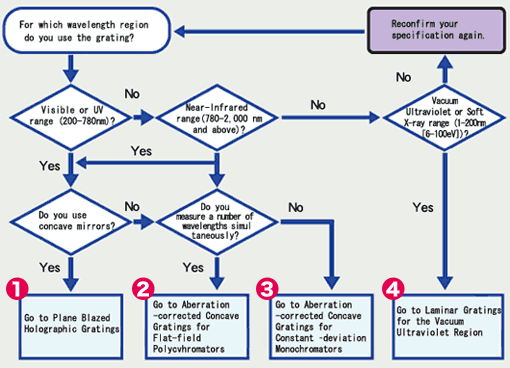Contents

## 5-1. Toroidal Diffraction Gratings

A toroidal diffraction grating is a sort of concave gratings. The toroidal shape is defined by two curvature radii at each central axis. Toroidal shapes where Rh is a horizontal curvature radius and Rv is a vertical curvature radius. The toroidal shapes are expressed in the following equation.

(14)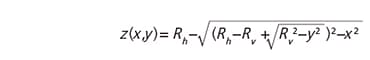The paraxial focal positions of a toroidal grating in Fig.9 are defined by equations (15) and (16). Here r is the entrance length, r'h is the horizontal focal length, and r'v is the vertical focal length. α and β satisfy the grating equation (2)'.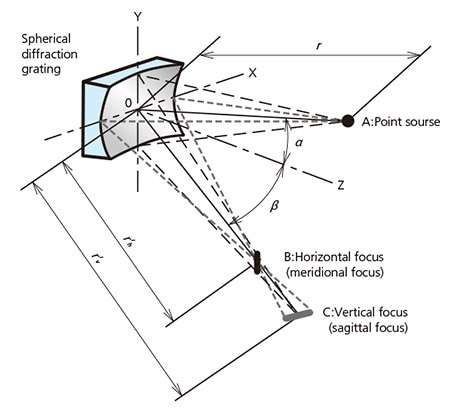Fig.9 The different focus of the Spherical diffraction grating

(15)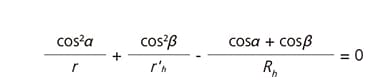(16)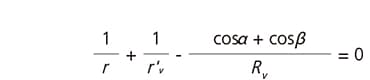The spherical diffraction gratings are formed on spherical blanks( Rh=Rv ) that has two focal lengths(r'hr'v ) has astigmatisms, The toroidal diffraction gratings are formed on toroidal (aspheric) blanks( RhRv ) that can correct astigmatisms.

## 5-2. Choice of a Grating

##### Selection Chart for Shimadzu Diffraction Gratings